# 基于三维建模的创意桌设计与实现Design and Implementation of Creative Table Based on 3D Modeling

DOI: 10.12677/AAM.2018.76084, PDF, 下载: 517  浏览: 809

Abstract: This paper proposes a set of circular folding table design based on three-dimensional modeling. When the folding table is propped up, the table top is round. When the table legs are flattened, the table top is a solid rectangle. Firstly, the length and width of the rectangle and the height of the ta-ble are set. The design scheme analyzes the specific scheme of the folding table by setting up the mathematical model. This process includes the design of the processing parameters and the mathematical description of the edge line of the table leg. Moreover, MATLAB is used to simulate the dynamic change of the folding table. Then, a mathematical model is established as for arbitrary rectangle length, width and table height, and an optimal design scheme is given which can design table with good stability and saving material. Ultimately, a more practical, more beautiful, and more innovative folding table is designed.

1. 引言

2. 折叠桌总方案

3. 限定条件模型

3.1. 模型建立

3.1.1. 确定滑槽模型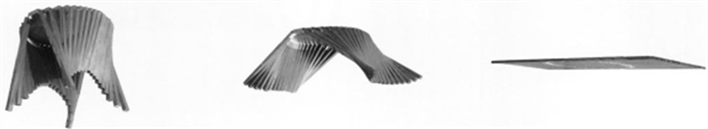Figure 1. Folding table image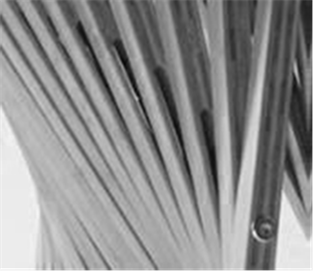Figure 2. The steel bar and the empty slot position schematic diagram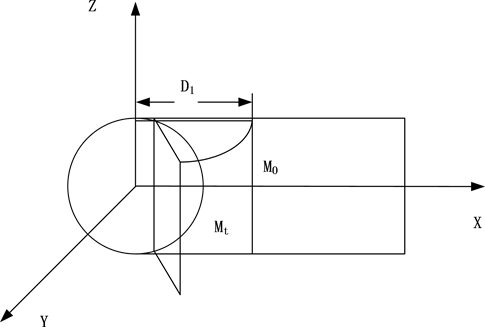Figure 3. Model diagram

$S:\left\{\begin{array}{l}x=\left({D}_{1}-a\right)\mathrm{cos}t+a\\ y=\left({D}_{1}-a\right)\mathrm{sin}t\end{array}$

${M}_{t}:\left\{\begin{array}{l}x-a=\left({D}_{1}-a\right)\mathrm{cos}t\\ y=\left({D}_{1}-a\right)\mathrm{sin}t\\ z=z\end{array}$

${M}^{\prime }{M}_{t}=\left(\left({D}_{1}-a\right)\mathrm{cos}t+a-{x}^{\prime },\left({D}_{1}-a\right)\mathrm{sin}t,0\right)$

${{x}^{\prime }}^{2}+{z}^{2}={r}^{2}$ (r为桌面半径)

${L}_{n}=\mathrm{max}|{M}^{\prime }{M}_{t}|-\mathrm{min}|{M}^{\prime }{M}_{t}|\text{\hspace{0.17em}}\left(1\le n\le 10\right)$

3.1.2. 确定桌脚模型

${M}^{\prime }M=k{M}^{\prime }{M}_{t}$

${M}^{\prime }{M}_{t}=\left(k\left(\left({D}_{1}-a\right)\mathrm{cos}t+a-{x}^{\prime }\right),k\left({D}_{1}-a\right)\mathrm{sin}t,0\right)$

${M}^{\prime }M=\left(x-{x}^{\prime },y,z\right)$

$\left\{\begin{array}{l}x-{x}^{\prime }=k\left[\left({D}_{1}-a\right)\mathrm{cos}t+a-{x}^{\prime }\right]\\ y=k\left({D}_{1}-a\right)\mathrm{sin}t\end{array}$

$\frac{y}{x-{x}^{\prime }}=\frac{\left({D}_{1}-a\right)\mathrm{sin}t}{\left({D}_{1}-a\right)\mathrm{cos}t+a-{x}^{\prime }}$

$\left\{\begin{array}{l}x=\sqrt{{r}^{2}-{z}^{2}}+k\left[\left({D}_{1}-a\right)\mathrm{cos}t-\sqrt{{r}^{2}-{z}^{2}}\right]\\ y=k\left({D}_{1}-a\right)\mathrm{sin}t\\ z=0\end{array}$

3.2. 模型求解

${x}^{\prime }=\sqrt{{r}^{2}-{z}^{2}}=\sqrt{{r}^{2}-{\left[\left(10-n\right)d\right]}^{2}}$

$a=\sqrt{{r}^{2}-{\left(r-d\right)}^{2}}=7.8\text{\hspace{0.17em}}\text{cm}$

${D}_{1}=\frac{60-a}{2}+a=33.9\text{\hspace{0.17em}}\text{cm}$

$\mathrm{sin}t=\frac{50}{60-a}=0.958$

3.3. MATLAB仿真

4. 不限条件模型

4.1. 模型建立

4.1.1. 确定滑槽模型

$S:\left\{\begin{array}{l}x=\left({D}_{1}-a\right)\mathrm{cos}t+a\\ y=\left({D}_{1}-a\right)\mathrm{sin}t\\ z=z\end{array}$

${M}_{t}:\left\{\begin{array}{l}x-a=\left({D}_{1}-a\right)\mathrm{cos}t\\ y=\left({D}_{1}-a\right)\mathrm{sin}t\\ z=z\end{array}$

${M}^{\prime }{M}_{t}=\left(\left({D}_{1}-a\right)\mathrm{cos}t+a-{x}^{\prime },\left({D}_{1}-a\right)\mathrm{sin}t,0\right)$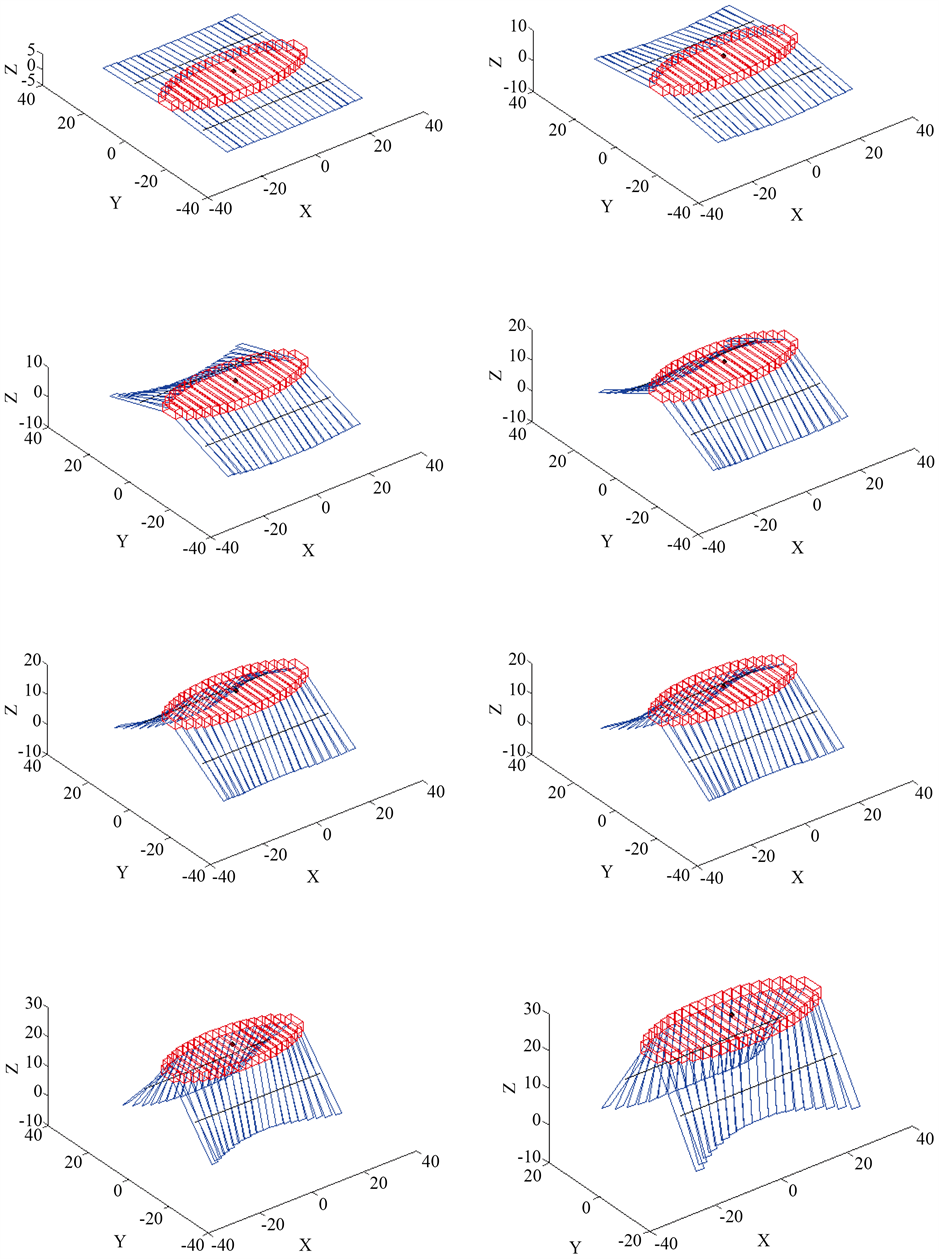Figure 4. Dynamic effect diagram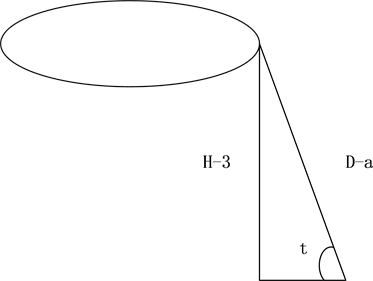Figure 5. Leg-ground intersection diagram

$\mathrm{sin}t=\frac{H-3}{\frac{L}{2}-a}$

$0\le t\le {t}_{0}$ ，则：

${t}_{0}=\mathrm{arcsin}\frac{H-3}{\frac{L}{2}-a}$

$0\le t\le \mathrm{arcsin}\frac{H-3}{\frac{L}{2}-a}$

$\partial \left(t\right)=|{M}^{\prime }{M}_{t}|=\sqrt{{\left({D}_{1}-a\right)}^{2}-2\ast \left({D}_{1}-a\right)\left({x}^{\prime }-a\right)\mathrm{cos}t+{\left({x}^{\prime }-a\right)}^{2}}$

${v}_{i}=\partial \left({t}_{0}\right)=\sqrt{{\left({D}_{1}-a\right)}^{2}-2\ast \left({D}_{1}-a\right)\left(f\left({z}_{i}\right)-a\right)\mathrm{cos}{t}_{0}+{\left(f\left({z}_{i}\right)-a\right)}^{2}}$

${u}_{i}=\partial \left(0\right)=\sqrt{{\left({D}_{1}-a\right)}^{2}-2\ast \left({D}_{1}-a\right)\left(f\left({z}_{i}\right)-a\right)+{\left(f\left({z}_{i}\right)-a\right)}^{2}}$

${L}_{p}={v}_{i}-{u}_{i}$

${z}_{1}=\frac{1}{2}d,{z}_{2}=\frac{1}{2}d+d,\cdots ,{z}_{i}=\frac{1}{2}a+\left(i-1\right)d$Figure 6. Schematic diagram when laying flatFigure 7. Table leg schematic diagram

4.1.2. 确定桌脚模型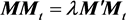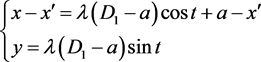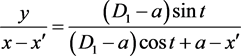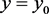，得到截痕方程：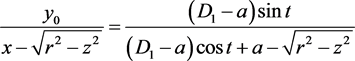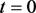时，曲面即为平面；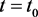时，得到曲面：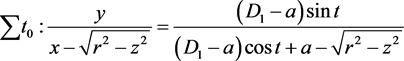4.2. 目标优化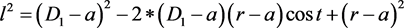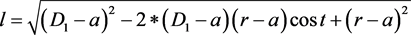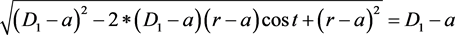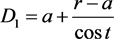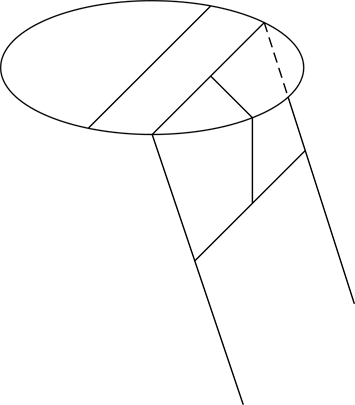Figure 8. Schematic diagram of stereogram

5. 总结

NOTES

*通讯作者。

  董晴. 对高等数学建模最优化理论的探究[J]. 科技资讯, 2015(25): 236-237.  秦小莉. 数学建模中的最优化理论探讨[J]. 剑南文学, 2010(5): 143.  于庆年. 数学与数学建模[J]. 辽宁财专学报, 2001(6): 53-55.  姜启源. 数学实验与数学建模[J]. 数学的实践与认识, 2001(5): 613-617.  郭文艳, 赵凤群. 数学建模及Matlab软件在矩阵运算教学中的应用[J]. 大学数学, 2013(4): 87-90.  李晓非. 数学建模实验与数学创新能力的培养[J]. 内江科技, 2005(4).  于润伟. Matlab基础及应用[M]. 北京: 机械工业出版社, 2010.  刘奕麟, 霍彪, 刘昱. MATLAB软件在数学建模中的应用[J]. 信息与电脑, 2016(17): 110-111.  吴新华. Matlab软件在数学建模中的应用[J]. 萍乡高等专科学校学报, 2012(3): 67-70.  郭文艳, 赵凤群. 数学建模及Matlab软件在矩阵运算教学中的应用[J]. 大学数学, 2013(4): 87-90.  徐秀华. Matlab软件在数学建模中的应用[J]. 科技与生活, 2010(13): 160-160, 155.  魏春艳, 郎晓林. MATLAB在数学建模中的应用[J]. 洛阳师范学院学报, 2007(5).  张琎. 浅谈应用Matlab的数学建模技术[J]. 福建电脑, 2005(10): 59-60.  张嘉炜. Matlab在数学建模中的应用[J]. 课程教育研究, 2017(4): 219.  华颖. MATLAB软件在数学建模中的应用[J]. 价值工程, 2013(26).